# Lord Ram

When lord Ram founded the breed white sheep was 8 more than black. Currently white sheep are four times higher than at the beginning and black three times more than at the beginning. White sheep is now 42 more than the black. How many white and black sheep together has Mr. Beran now?

x =  102

### Step-by-step explanation:

x = b+c
b = 42+c
b = 4a
c = 3(a-8)

x = b+c
b = 42+c
b = 4•a
c = 3•(a-8)

b+c-x = 0
b-c = 42
4a-b = 0
3a-c = 24

a = 18
b = 72
c = 30
x = 102

Our linear equations calculator calculates it.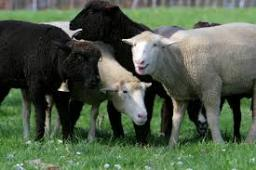Did you find an error or inaccuracy? Feel free to write us. Thank you!Tips to related online calculators
Do you have a system of equations and looking for calculator system of linear equations?

## Related math problems and questions:On the meadow grazing horses, cows, and sheep, together with less than 200. If cows were 45 times more, horses 60 times more, and sheep 35 times more than there are now, their numbers would equally. How many horses, cows, and sheep are on the meadow toget
• BW-BS balls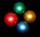Adam has a full box of balls that are large or small, black or white. Ratio of large and small balls is 5:3. Within the large balls the ratio of the black to white is 1:2 and between small balls the ratio of the black to white is 1:8 What is the ratio of
• Chamber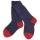In the chamber light is broken and all from it must be taken at random. Socks have four different colors. If you want to be sure of pulling at least two white socks, we have to bring them out 28 from the chamber. In order to have such certainty for the pa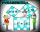Two fifth-graders teams competing in math competitions - in Mathematical Olympiad and Pytagoriade. Of the 33 students competed in at least one of the contest 22 students. Students who competed only in Pytagoriade were twice more than those who just compet
• Shepherd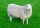Kuba makes a deal with a shepherd to take care of his sheep. Shepherd said to Kuba that he would receive twenty gold coins and one sheep after a year of service. But Kuba resigned just after the seventh month of service. But shepherd rewarded him and paid
• Jane and Miro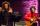Jane's brother Miro is 42 years. And he is three times old as it was Jane when Miro was for so many years as there are now Jane. How old is Jane?
• Beds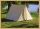At the summer camp, there are 41 chalets. Some rooms are 3-beds, some 4-beds. How many campers from 140 are living in 3-bed?
• Scouts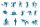Three scouts went on a three day trip. The second day went 4 km more than the first day. The third day went two times less than the first day. They went along 54 km. How many kilometers went every day together?
• Tea blendTea blends are maked from two kinds of tea. In standard tea mixture are two teas in the ratio 1:3 and 40 g costs 42 CZK. In the premium tea mixture are weighing two teas in the ratio 1:1 and 50 grams costs 60 CZK. How much cost 10 grams of more expensive
• Z9–I–4 MO 2017Numbers 1, 2, 3, 4, 5, 6, 7, 8 and 9 were prepared for a train journey with three wagons. They wanted to sit out so that three numbers were seated in each carriage and the largest of each of the three was equal to the sum of the remaining two. The conduct
• Sheep and cowsThere are only sheep and cows on the farm. Sheep is eight more than cows. The number of cows is half the number of sheep. How many animals live on the farm?
• White and black ballsThere are 7 white and 3 black balls in an opaque pocket. The balls are the same size. a) Randomly pull out one ball. What is the probability that it will be white? We pull out one ball, see what color it is and return it to the pocket. Then we pull out th
• Lookout towerHow high is the lookout tower? If each step was 3 cm lower, 60 more of them were on the lookout tower. If it were 3 cm higher again, it would be 40 less than it is now.
• Star equationWrite digits instead of stars so that the sum of the written digits is odd and is true equality: 42 · ∗8 = 2 ∗∗∗
• Last digitWhat is the last number of 2016 power of 2017
• Rabbits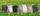In the hutch are 48 mottled rabbits. Brown are 23 less than mottled and white are 8-times less than mottled. How many rabbits are in the hutch?
• MO Z8-I-1 2018Fero and David meet daily in the elevator. One morning they found that if they multiply their current age, they get 238. If they did the same after four years, this product would be 378. Determine the sum of the current ages of Fero and David.